# Selina Solutions Concise Maths Class 7 Chapter 2: Rational Numbers Exercise 2C

Selina Solutions Concise Maths Class 7 Chapter 2 Rational Numbers Exercise 2C has problems on the addition and subtraction of rational numbers, along with various cases. Students are advised to practice the exercise wise problems on a daily basis to improve their understanding of the concepts. PDF of solutions can be downloaded by the students either chapter wise or exercise wise, based on their needs. Students can cross check their answers and understand the different methods of solving problems using PDF. Selina Solutions Concise Maths Class 7 Chapter 2 Rational Numbers Exercise 2C PDF is provided below, for free download.

## Selina Solutions Concise Maths Class 7 Chapter 2: Rational Numbers Exercise 2C Download PDF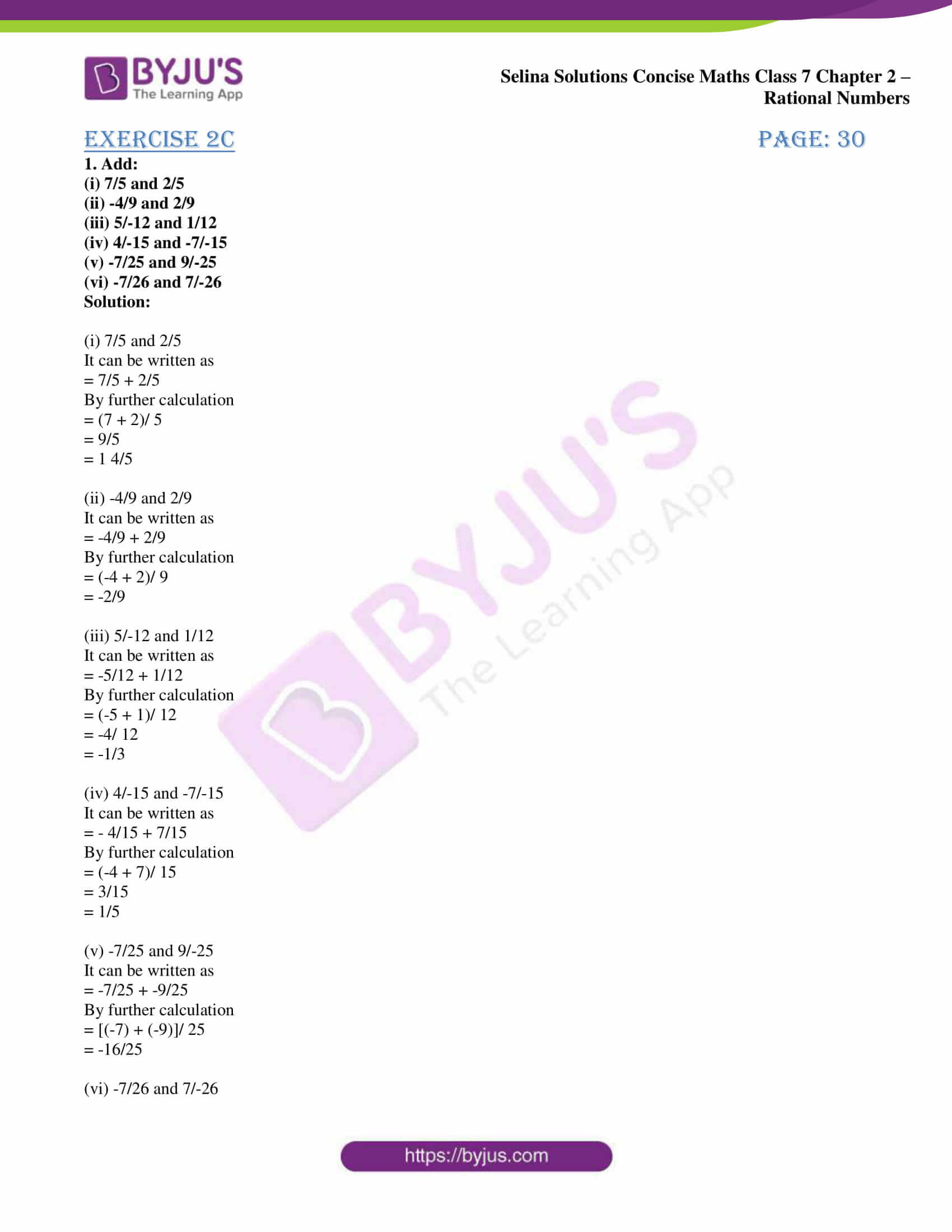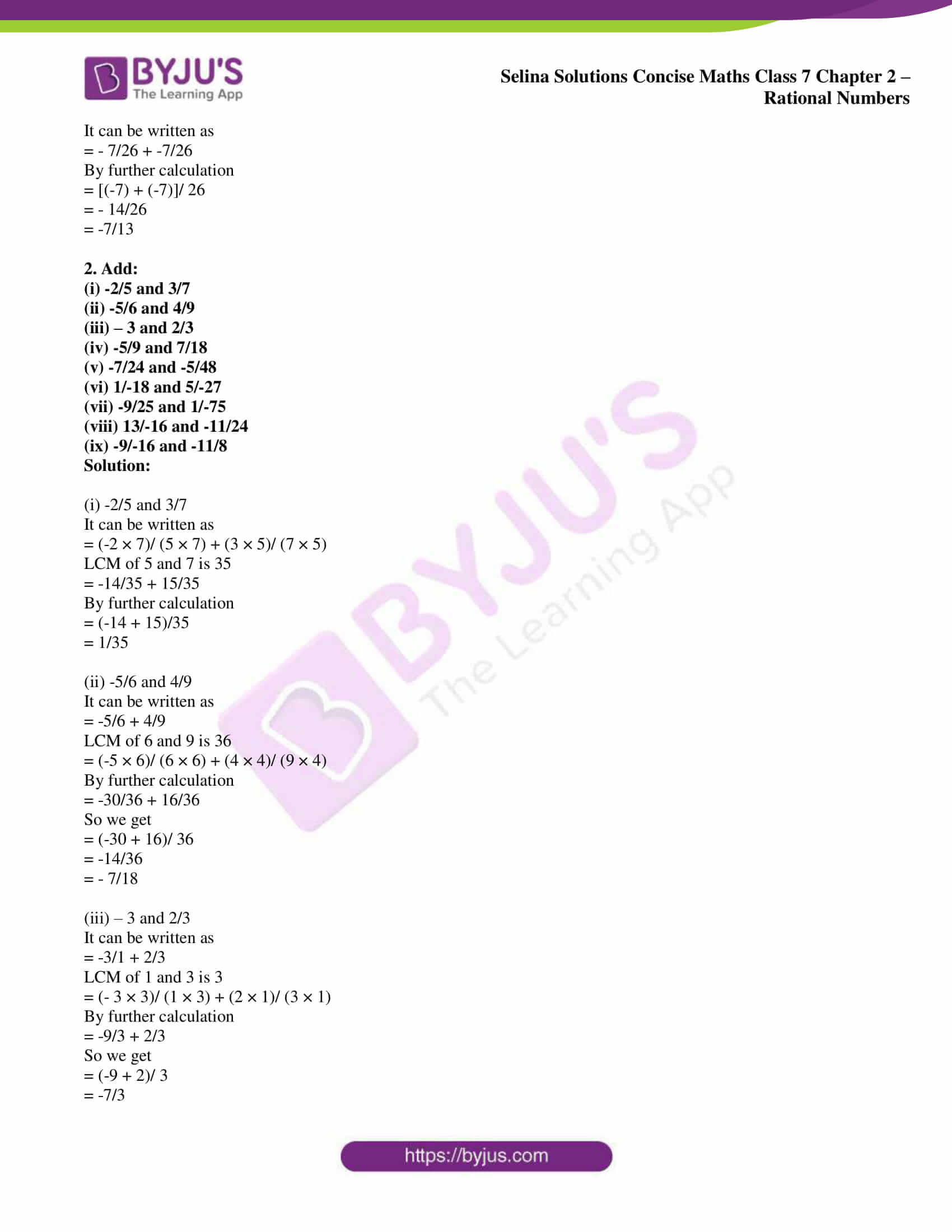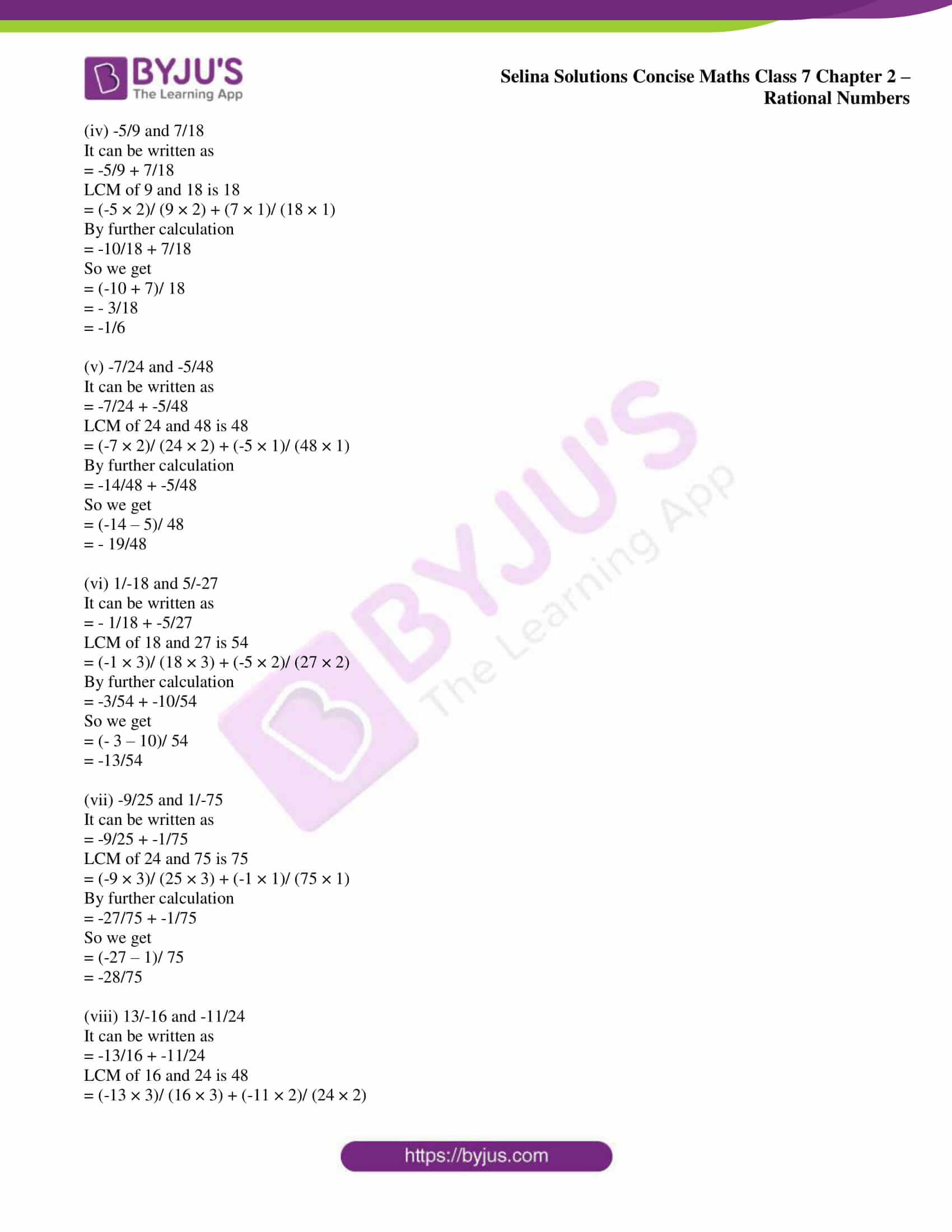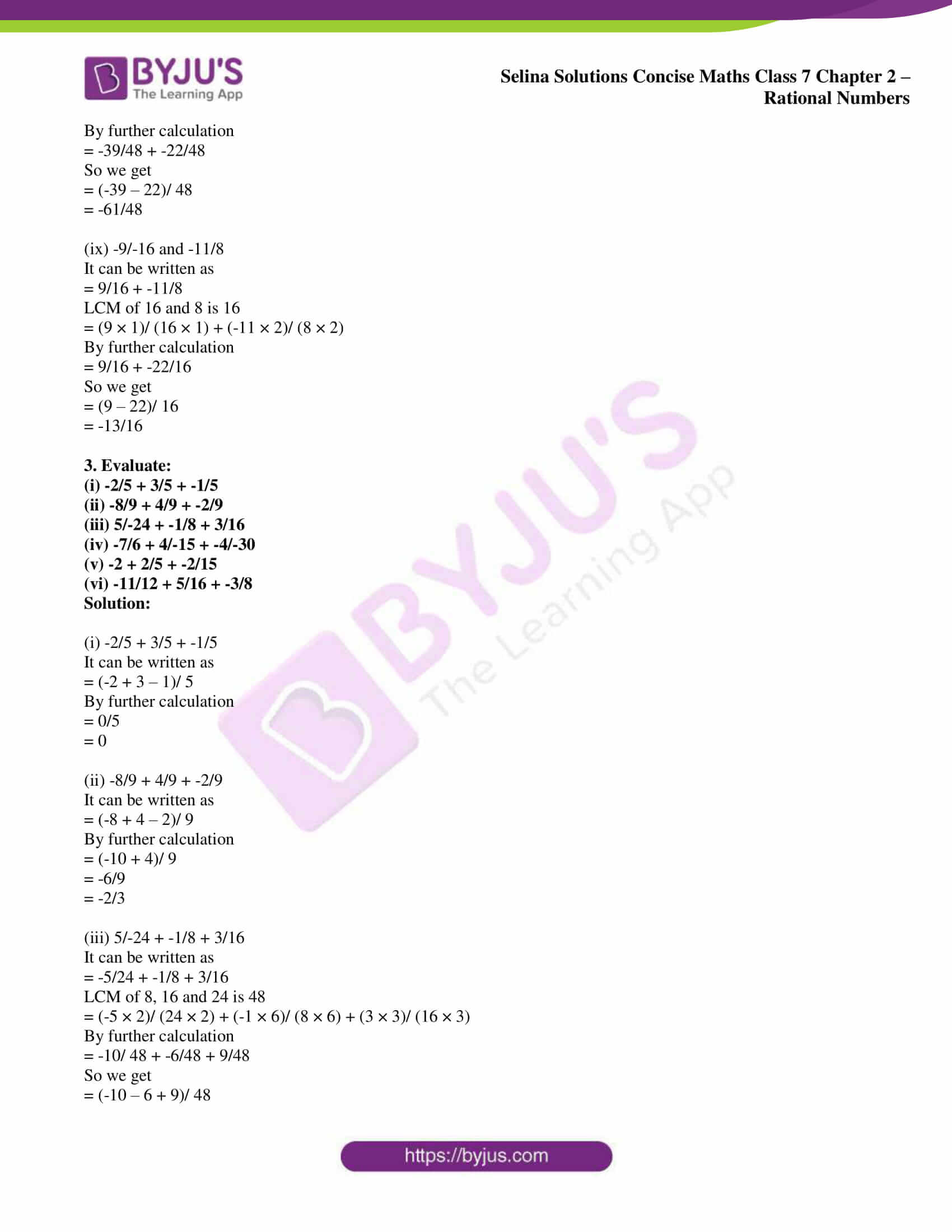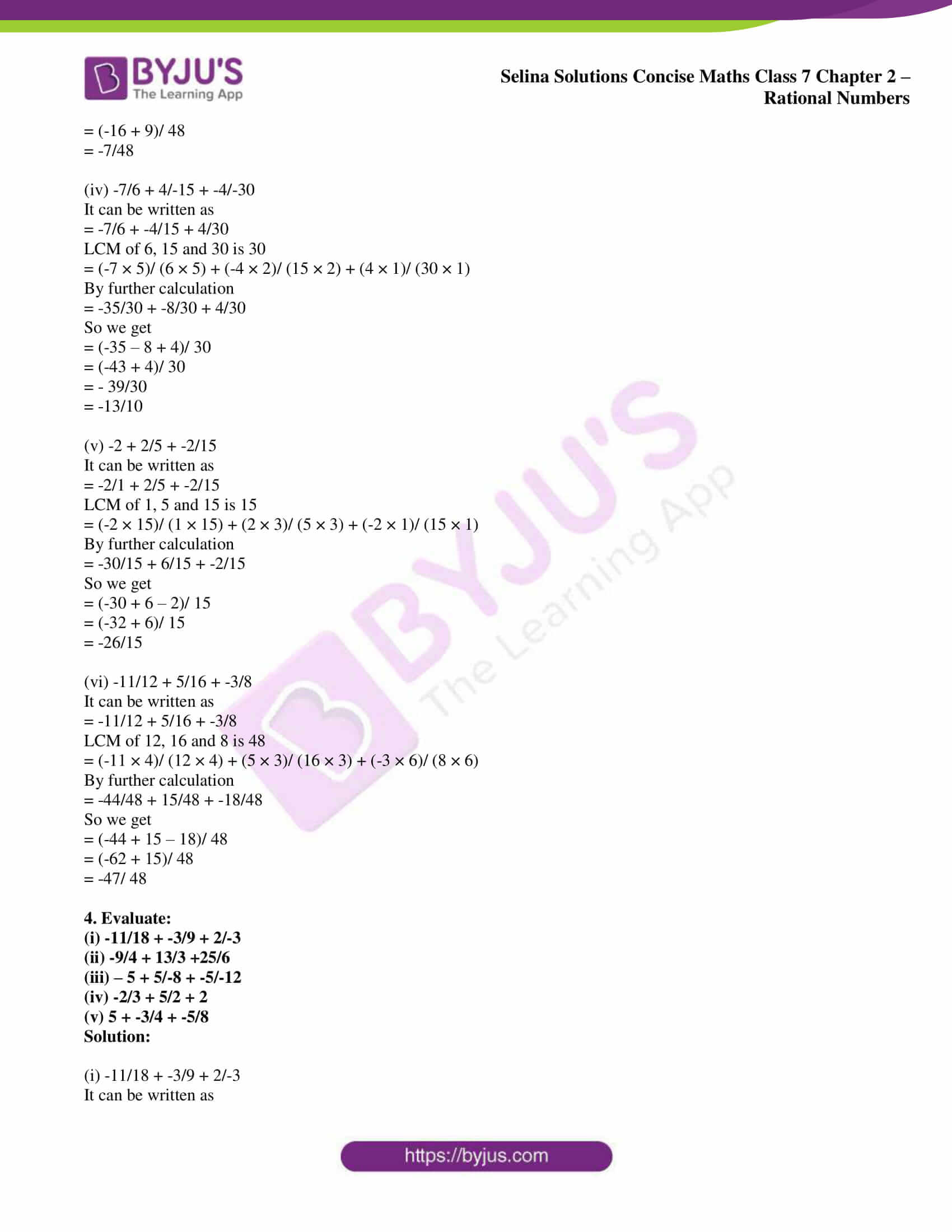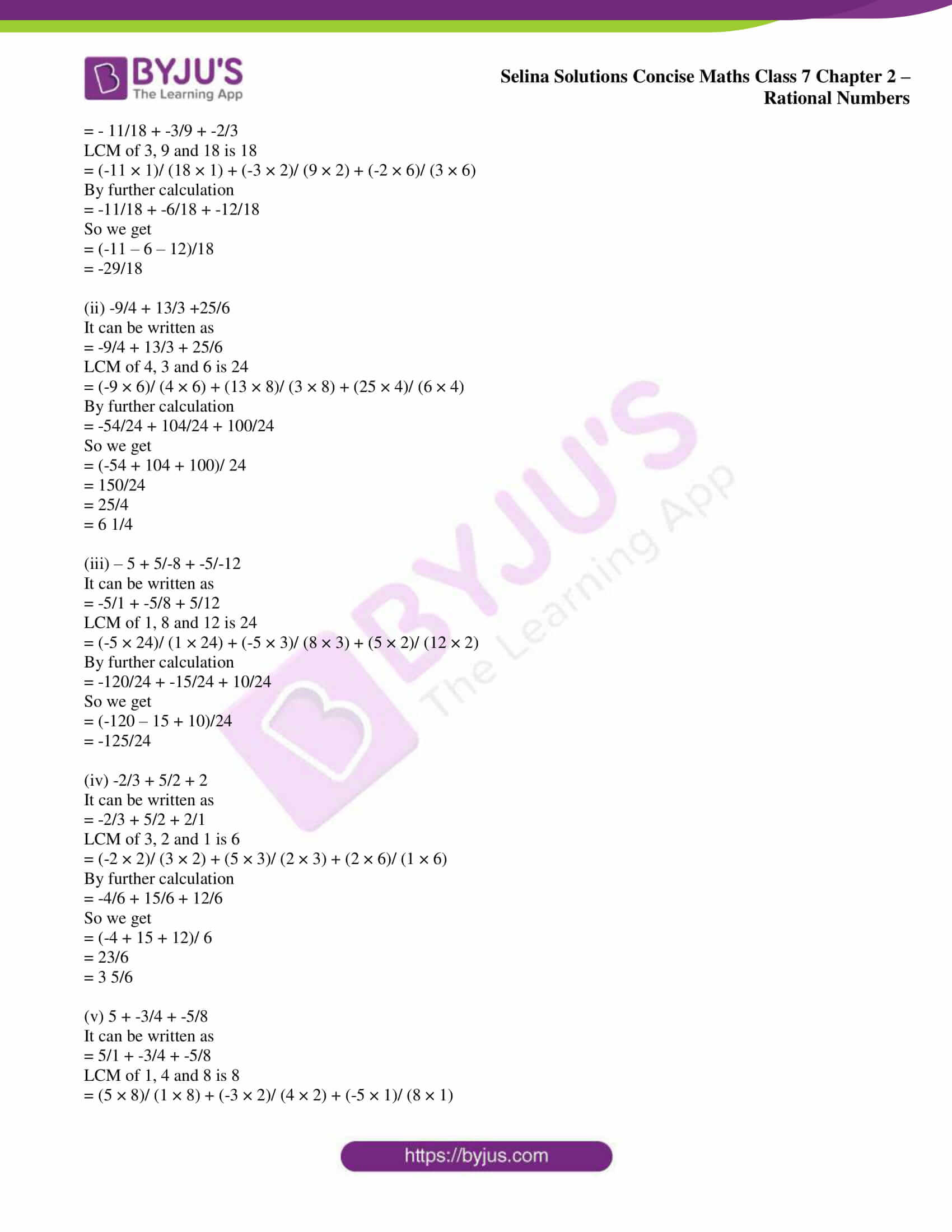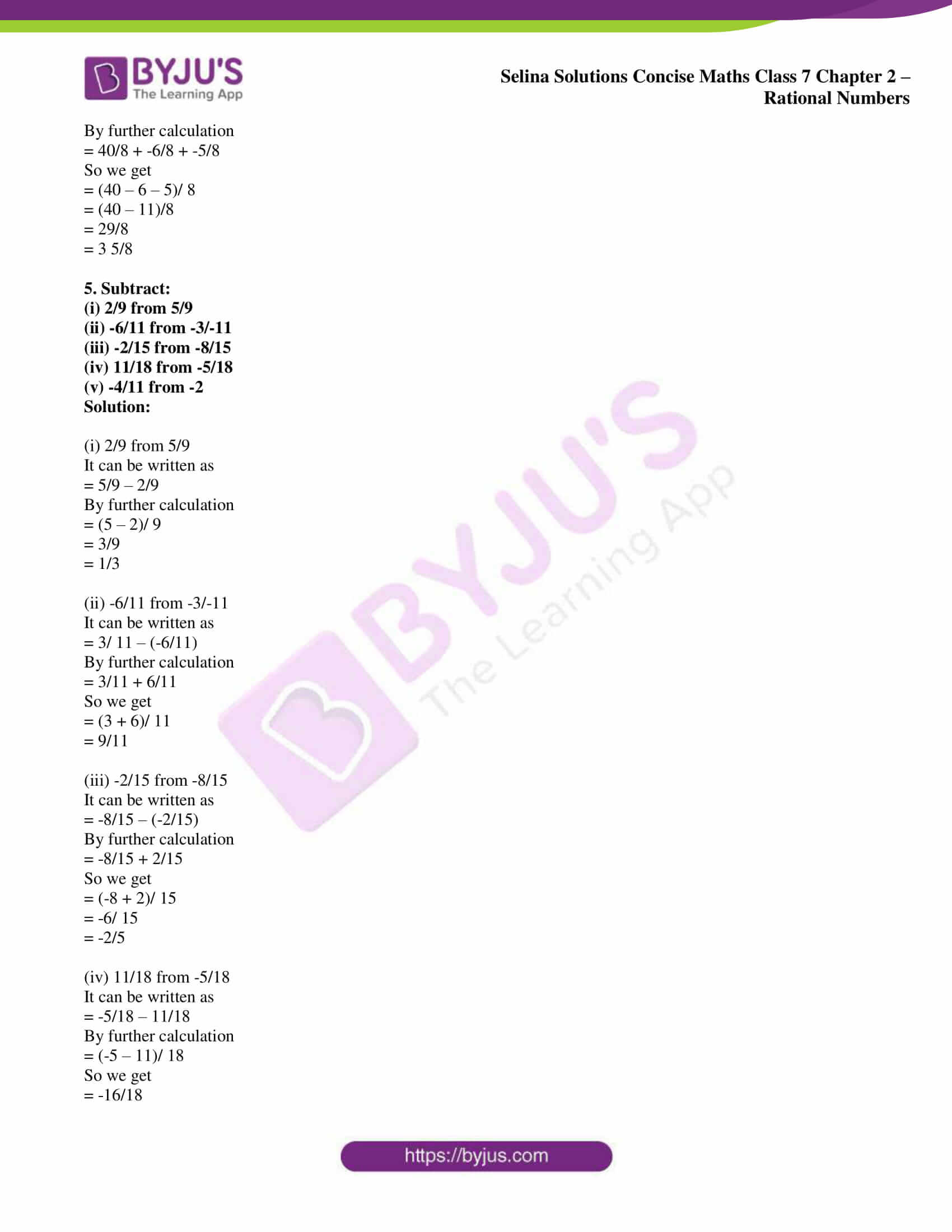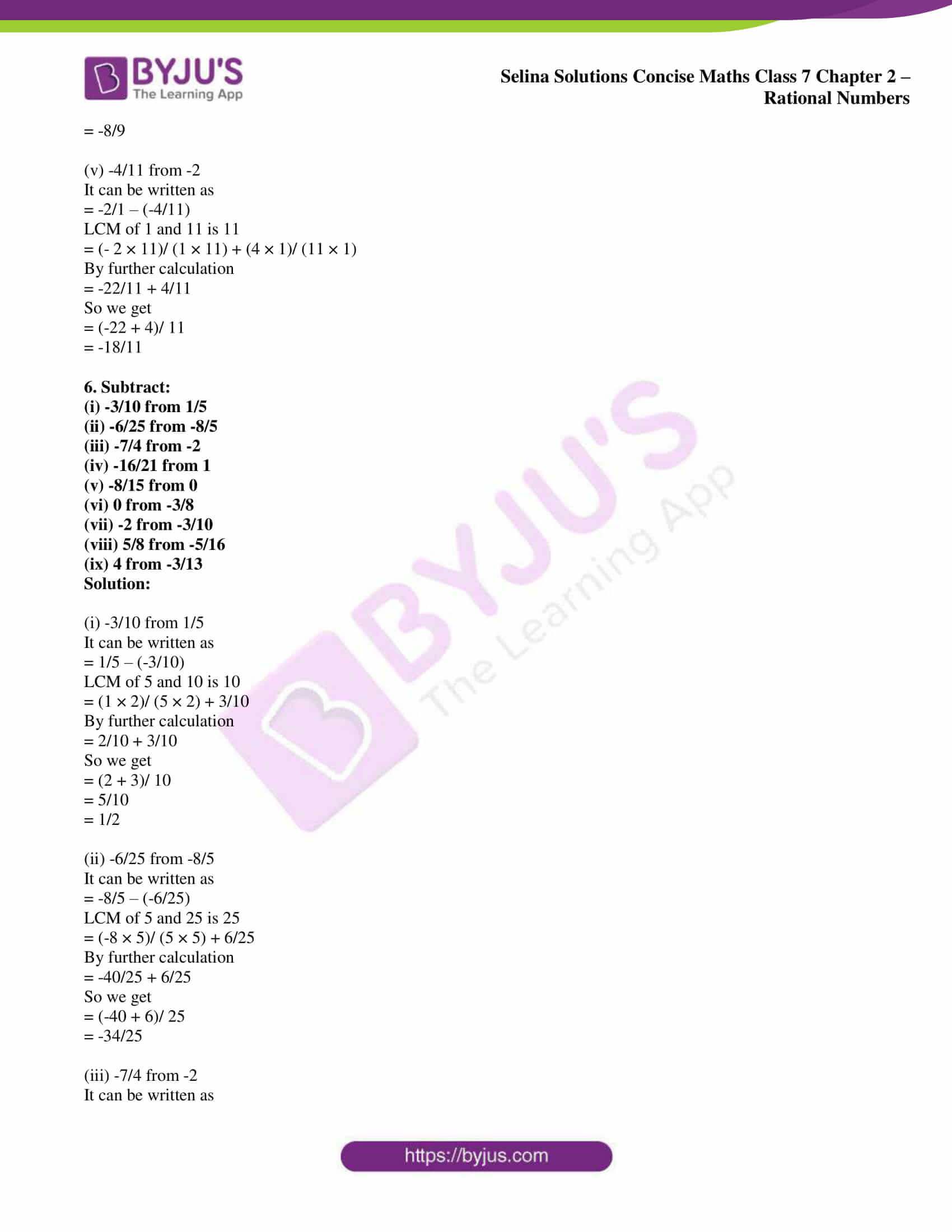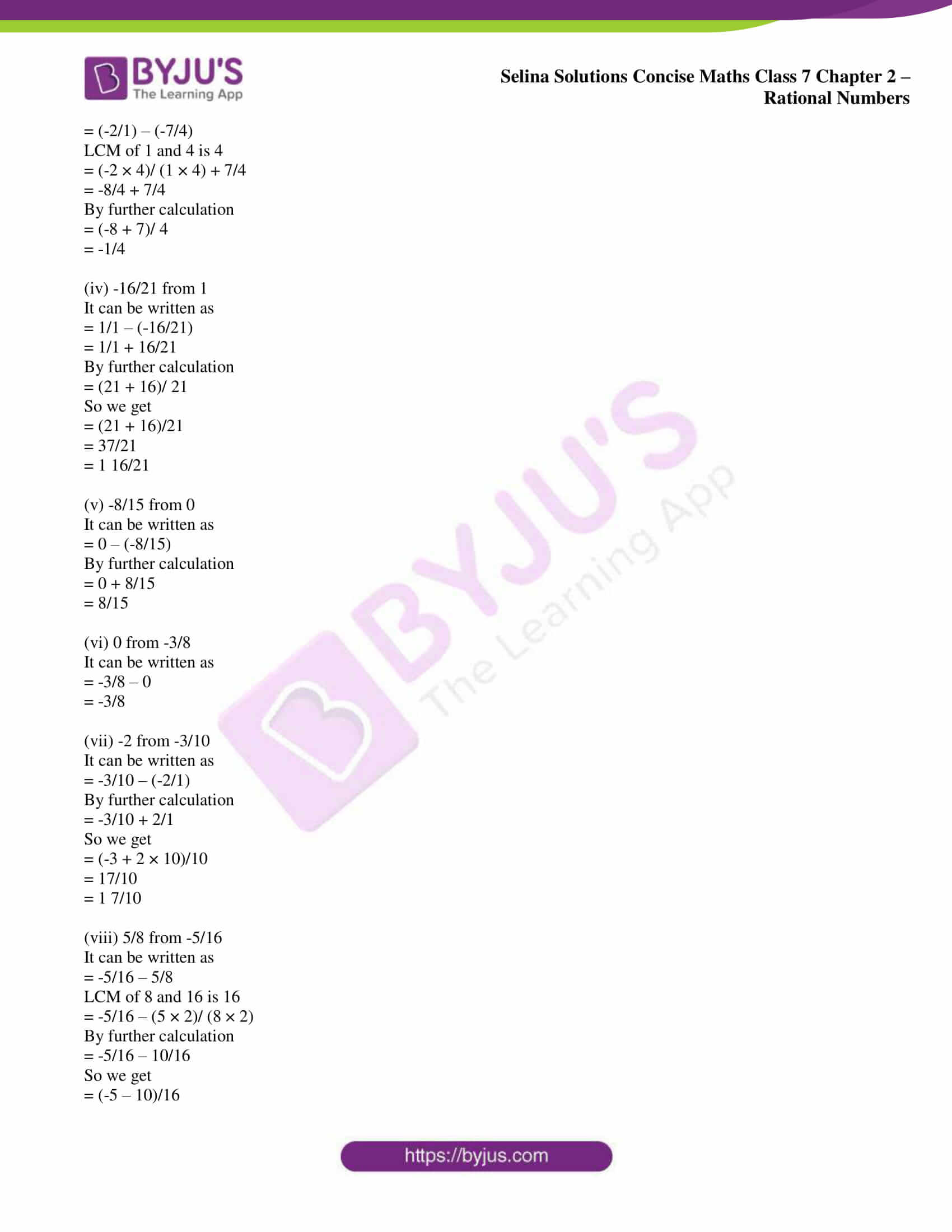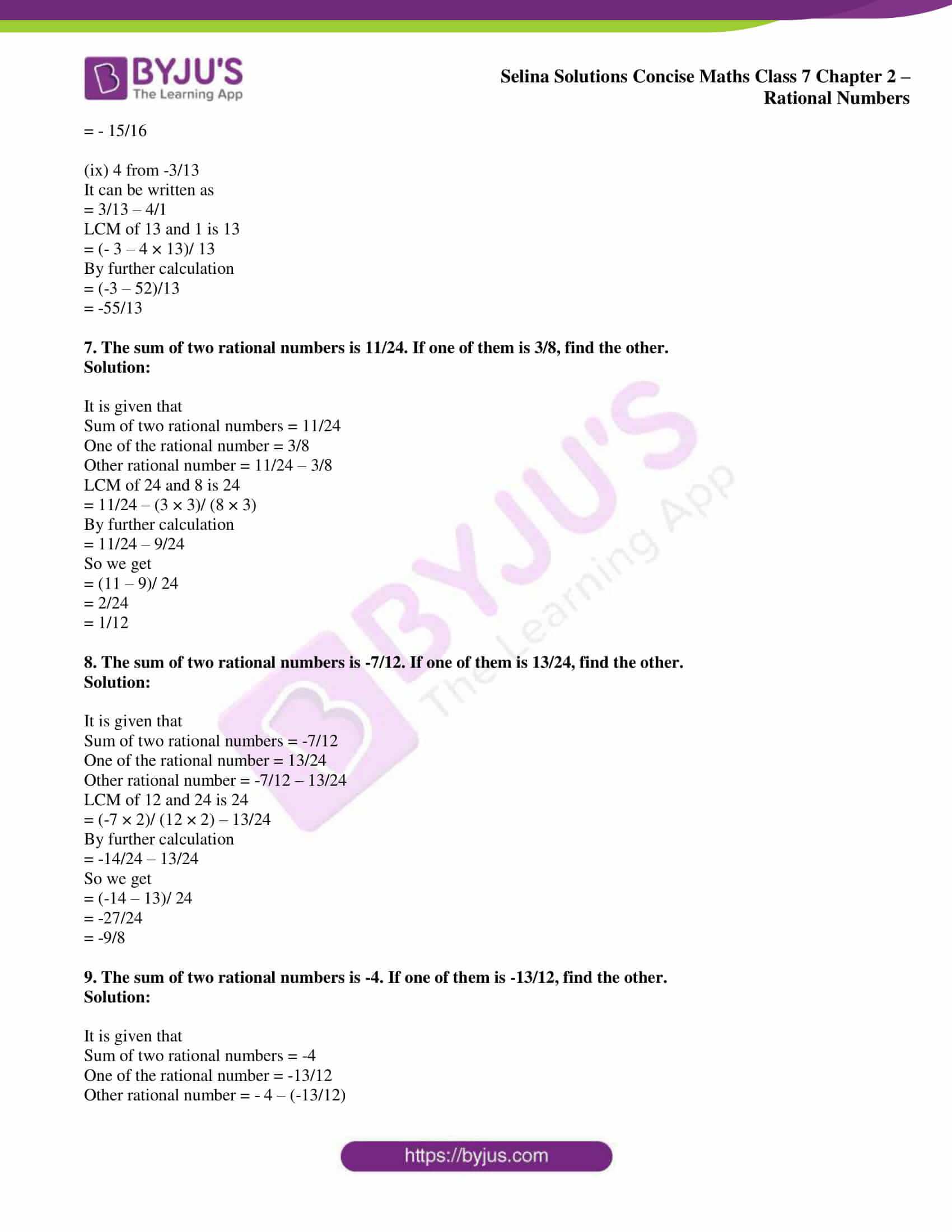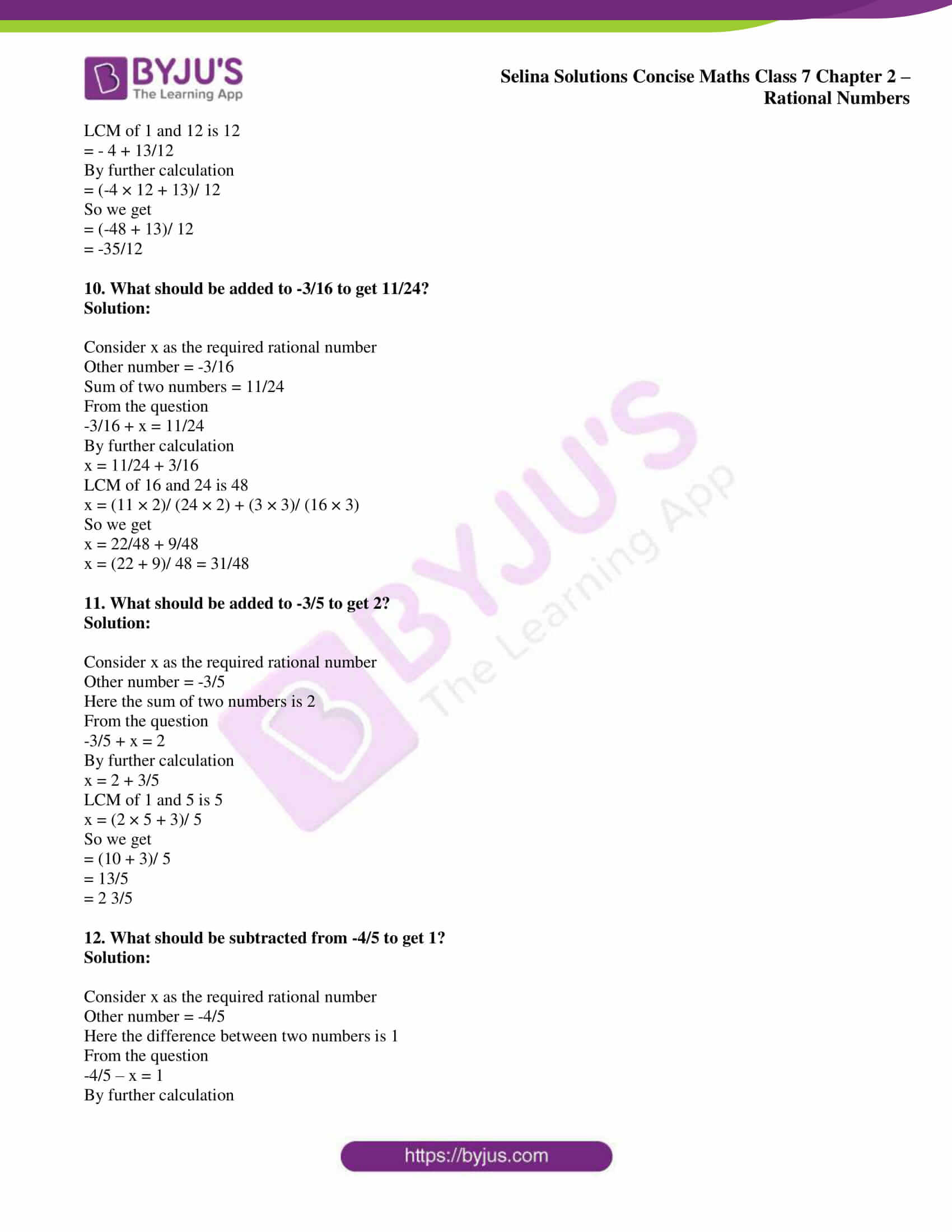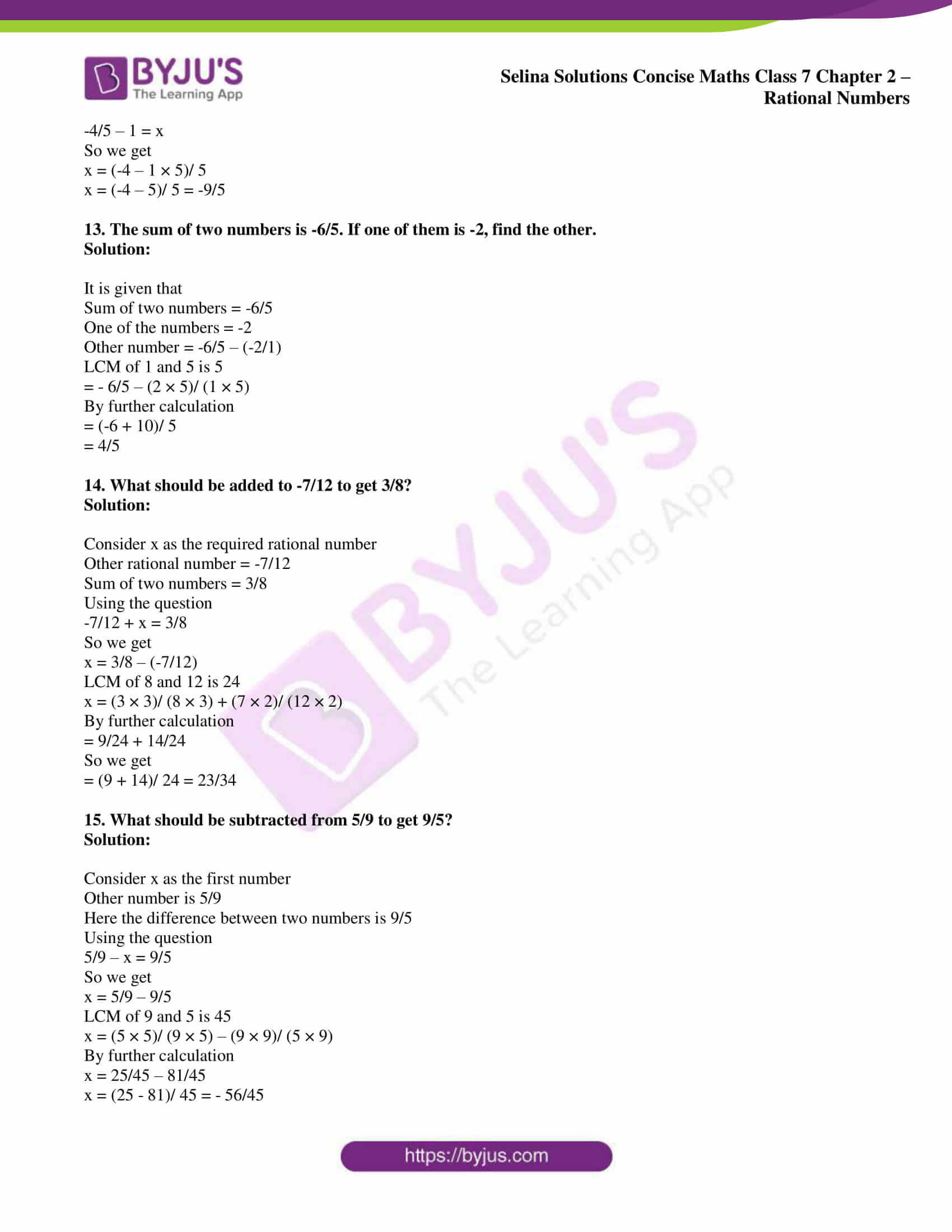### Access Selina Solutions Concise Maths Class 7 Chapter 2: Rational Numbers Exercise 2C

(i) 7/5 and 2/5

(ii) -4/9 and 2/9

(iii) 5/-12 and 1/12

(iv) 4/-15 and -7/-15

(v) -7/25 and 9/-25

(vi) -7/26 and 7/-26

Solution:

(i) 7/5 and 2/5

It can be written as

= 7/5 + 2/5

By further calculation

= (7 + 2)/ 5

= 9/5

= 1 4/5

(ii) -4/9 and 2/9

It can be written as

= -4/9 + 2/9

By further calculation

= (-4 + 2)/ 9

= -2/9

(iii) 5/-12 and 1/12

It can be written as

= -5/12 + 1/12

By further calculation

= (-5 + 1)/ 12

= -4/ 12

= -1/3

(iv) 4/-15 and -7/-15

It can be written as

= – 4/15 + 7/15

By further calculation

= (-4 + 7)/ 15

= 3/15

= 1/5

(v) -7/25 and 9/-25

It can be written as

= -7/25 + -9/25

By further calculation

= [(-7) + (-9)]/ 25

= -16/25

(vi) -7/26 and 7/-26

It can be written as

= – 7/26 + -7/26

By further calculation

= [(-7) + (-7)]/ 26

= – 14/26

= -7/13

(i) -2/5 and 3/7

(ii) -5/6 and 4/9

(iii) – 3 and 2/3

(iv) -5/9 and 7/18

(v) -7/24 and -5/48

(vi) 1/-18 and 5/-27

(vii) -9/25 and 1/-75

(viii) 13/-16 and -11/24

(ix) -9/-16 and -11/8

Solution:

(i) -2/5 and 3/7

It can be written as

= (-2 × 7)/ (5 × 7) + (3 × 5)/ (7 × 5)

LCM of 5 and 7 is 35

= -14/35 + 15/35

By further calculation

= (-14 + 15)/35

= 1/35

(ii) -5/6 and 4/9

It can be written as

= -5/6 + 4/9

LCM of 6 and 9 is 36

= (-5 × 6)/ (6 × 6) + (4 × 4)/ (9 × 4)

By further calculation

= -30/36 + 16/36

So we get

= (-30 + 16)/ 36

= -14/36

= – 7/18

(iii) – 3 and 2/3

It can be written as

= -3/1 + 2/3

LCM of 1 and 3 is 3

= (- 3 × 3)/ (1 × 3) + (2 × 1)/ (3 × 1)

By further calculation

= -9/3 + 2/3

So we get

= (-9 + 2)/ 3

= -7/3

(iv) -5/9 and 7/18

It can be written as

= -5/9 + 7/18

LCM of 9 and 18 is 18

= (-5 × 2)/ (9 × 2) + (7 × 1)/ (18 × 1)

By further calculation

= -10/18 + 7/18

So we get

= (-10 + 7)/ 18

= – 3/18

= -1/6

(v) -7/24 and -5/48

It can be written as

= -7/24 + -5/48

LCM of 24 and 48 is 48

= (-7 × 2)/ (24 × 2) + (-5 × 1)/ (48 × 1)

By further calculation

= -14/48 + -5/48

So we get

= (-14 – 5)/ 48

= – 19/48

(vi) 1/-18 and 5/-27

It can be written as

= – 1/18 + -5/27

LCM of 18 and 27 is 54

= (-1 × 3)/ (18 × 3) + (-5 × 2)/ (27 × 2)

By further calculation

= -3/54 + -10/54

So we get

= (- 3 – 10)/ 54

= -13/54

(vii) -9/25 and 1/-75

It can be written as

= -9/25 + -1/75

LCM of 24 and 75 is 75

= (-9 × 3)/ (25 × 3) + (-1 × 1)/ (75 × 1)

By further calculation

= -27/75 + -1/75

So we get

= (-27 – 1)/ 75

= -28/75

(viii) 13/-16 and -11/24

It can be written as

= -13/16 + -11/24

LCM of 16 and 24 is 48

= (-13 × 3)/ (16 × 3) + (-11 × 2)/ (24 × 2)

By further calculation

= -39/48 + -22/48

So we get

= (-39 – 22)/ 48

= -61/48

(ix) -9/-16 and -11/8

It can be written as

= 9/16 + -11/8

LCM of 16 and 8 is 16

= (9 × 1)/ (16 × 1) + (-11 × 2)/ (8 × 2)

By further calculation

= 9/16 + -22/16

So we get

= (9 – 22)/ 16

= -13/16

3. Evaluate:

(i) -2/5 + 3/5 + -1/5

(ii) -8/9 + 4/9 + -2/9

(iii) 5/-24 + -1/8 + 3/16

(iv) -7/6 + 4/-15 + -4/-30

(v) -2 + 2/5 + -2/15

(vi) -11/12 + 5/16 + -3/8

Solution:

(i) -2/5 + 3/5 + -1/5

It can be written as

= (-2 + 3 – 1)/ 5

By further calculation

= 0/5

= 0

(ii) -8/9 + 4/9 + -2/9

It can be written as

= (-8 + 4 – 2)/ 9

By further calculation

= (-10 + 4)/ 9

= -6/9

= -2/3

(iii) 5/-24 + -1/8 + 3/16

It can be written as

= -5/24 + -1/8 + 3/16

LCM of 8, 16 and 24 is 48

= (-5 × 2)/ (24 × 2) + (-1 × 6)/ (8 × 6) + (3 × 3)/ (16 × 3)

By further calculation

= -10/ 48 + -6/48 + 9/48

So we get

= (-10 – 6 + 9)/ 48

= (-16 + 9)/ 48

= -7/48

(iv) -7/6 + 4/-15 + -4/-30

It can be written as

= -7/6 + -4/15 + 4/30

LCM of 6, 15 and 30 is 30

= (-7 × 5)/ (6 × 5) + (-4 × 2)/ (15 × 2) + (4 × 1)/ (30 × 1)

By further calculation

= -35/30 + -8/30 + 4/30

So we get

= (-35 – 8 + 4)/ 30

= (-43 + 4)/ 30

= – 39/30

= -13/10

(v) -2 + 2/5 + -2/15

It can be written as

= -2/1 + 2/5 + -2/15

LCM of 1, 5 and 15 is 15

= (-2 × 15)/ (1 × 15) + (2 × 3)/ (5 × 3) + (-2 × 1)/ (15 × 1)

By further calculation

= -30/15 + 6/15 + -2/15

So we get

= (-30 + 6 – 2)/ 15

= (-32 + 6)/ 15

= -26/15

(vi) -11/12 + 5/16 + -3/8

It can be written as

= -11/12 + 5/16 + -3/8

LCM of 12, 16 and 8 is 48

= (-11 × 4)/ (12 × 4) + (5 × 3)/ (16 × 3) + (-3 × 6)/ (8 × 6)

By further calculation

= -44/48 + 15/48 + -18/48

So we get

= (-44 + 15 – 18)/ 48

= (-62 + 15)/ 48

= -47/ 48

4. Evaluate:

(i) -11/18 + -3/9 + 2/-3

(ii) -9/4 + 13/3 +25/6

(iii) – 5 + 5/-8 + -5/-12

(iv) -2/3 + 5/2 + 2

(v) 5 + -3/4 + -5/8

Solution:

(i) -11/18 + -3/9 + 2/-3

It can be written as

= – 11/18 + -3/9 + -2/3

LCM of 3, 9 and 18 is 18

= (-11 × 1)/ (18 × 1) + (-3 × 2)/ (9 × 2) + (-2 × 6)/ (3 × 6)

By further calculation

= -11/18 + -6/18 + -12/18

So we get

= (-11 – 6 – 12)/18

= -29/18

(ii) -9/4 + 13/3 +25/6

It can be written as

= -9/4 + 13/3 + 25/6

LCM of 4, 3 and 6 is 24

= (-9 × 6)/ (4 × 6) + (13 × 8)/ (3 × 8) + (25 × 4)/ (6 × 4)

By further calculation

= -54/24 + 104/24 + 100/24

So we get

= (-54 + 104 + 100)/ 24

= 150/24

= 25/4

= 6 1/4

(iii) – 5 + 5/-8 + -5/-12

It can be written as

= -5/1 + -5/8 + 5/12

LCM of 1, 8 and 12 is 24

= (-5 × 24)/ (1 × 24) + (-5 × 3)/ (8 × 3) + (5 × 2)/ (12 × 2)

By further calculation

= -120/24 + -15/24 + 10/24

So we get

= (-120 – 15 + 10)/24

= -125/24

(iv) -2/3 + 5/2 + 2

It can be written as

= -2/3 + 5/2 + 2/1

LCM of 3, 2 and 1 is 6

= (-2 × 2)/ (3 × 2) + (5 × 3)/ (2 × 3) + (2 × 6)/ (1 × 6)

By further calculation

= -4/6 + 15/6 + 12/6

So we get

= (-4 + 15 + 12)/ 6

= 23/6

= 3 5/6

(v) 5 + -3/4 + -5/8

It can be written as

= 5/1 + -3/4 + -5/8

LCM of 1, 4 and 8 is 8

= (5 × 8)/ (1 × 8) + (-3 × 2)/ (4 × 2) + (-5 × 1)/ (8 × 1)

By further calculation

= 40/8 + -6/8 + -5/8

So we get

= (40 – 6 – 5)/ 8

= (40 – 11)/8

= 29/8

= 3 5/8

5. Subtract:

(i) 2/9 from 5/9

(ii) -6/11 from -3/-11

(iii) -2/15 from -8/15

(iv) 11/18 from -5/18

(v) -4/11 from -2

Solution:

(i) 2/9 from 5/9

It can be written as

= 5/9 – 2/9

By further calculation

= (5 – 2)/ 9

= 3/9

= 1/3

(ii) -6/11 from -3/-11

It can be written as

= 3/ 11 – (-6/11)

By further calculation

= 3/11 + 6/11

So we get

= (3 + 6)/ 11

= 9/11

(iii) -2/15 from -8/15

It can be written as

= -8/15 – (-2/15)

By further calculation

= -8/15 + 2/15

So we get

= (-8 + 2)/ 15

= -6/ 15

= -2/5

(iv) 11/18 from -5/18

It can be written as

= -5/18 – 11/18

By further calculation

= (-5 – 11)/ 18

So we get

= -16/18

= -8/9

(v) -4/11 from -2

It can be written as

= -2/1 – (-4/11)

LCM of 1 and 11 is 11

= (- 2 × 11)/ (1 × 11) + (4 × 1)/ (11 × 1)

By further calculation

= -22/11 + 4/11

So we get

= (-22 + 4)/ 11

= -18/11

6. Subtract:

(i) -3/10 from 1/5

(ii) -6/25 from -8/5

(iii) -7/4 from -2

(iv) -16/21 from 1

(v) -8/15 from 0

(vi) 0 from -3/8

(vii) -2 from -3/10

(viii) 5/8 from -5/16

(ix) 4 from -3/13

Solution:

(i) -3/10 from 1/5

It can be written as

= 1/5 – (-3/10)

LCM of 5 and 10 is 10

= (1 × 2)/ (5 × 2) + 3/10

By further calculation

= 2/10 + 3/10

So we get

= (2 + 3)/ 10

= 5/10

= 1/2

(ii) -6/25 from -8/5

It can be written as

= -8/5 – (-6/25)

LCM of 5 and 25 is 25

= (-8 × 5)/ (5 × 5) + 6/25

By further calculation

= -40/25 + 6/25

So we get

= (-40 + 6)/ 25

= -34/25

(iii) -7/4 from -2

It can be written as

= (-2/1) – (-7/4)

LCM of 1 and 4 is 4

= (-2 × 4)/ (1 × 4) + 7/4

= -8/4 + 7/4

By further calculation

= (-8 + 7)/ 4

= -1/4

(iv) -16/21 from 1

It can be written as

= 1/1 – (-16/21)

= 1/1 + 16/21

By further calculation

= (21 + 16)/ 21

So we get

= (21 + 16)/21

= 37/21

= 1 16/21

(v) -8/15 from 0

It can be written as

= 0 – (-8/15)

By further calculation

= 0 + 8/15

= 8/15

(vi) 0 from -3/8

It can be written as

= -3/8 – 0

= -3/8

(vii) -2 from -3/10

It can be written as

= -3/10 – (-2/1)

By further calculation

= -3/10 + 2/1

So we get

= (-3 + 2 × 10)/10

= 17/10

= 1 7/10

(viii) 5/8 from -5/16

It can be written as

= -5/16 – 5/8

LCM of 8 and 16 is 16

= -5/16 – (5 × 2)/ (8 × 2)

By further calculation

= -5/16 – 10/16

So we get

= (-5 – 10)/16

= – 15/16

(ix) 4 from -3/13

It can be written as

= 3/13 – 4/1

LCM of 13 and 1 is 13

= (- 3 – 4 × 13)/ 13

By further calculation

= (-3 – 52)/13

= -55/13

7. The sum of two rational numbers is 11/24. If one of them is 3/8, find the other.

Solution:

It is given that

Sum of two rational numbers = 11/24

One of the rational number = 3/8

Other rational number = 11/24 – 3/8

LCM of 24 and 8 is 24

= 11/24 – (3 × 3)/ (8 × 3)

By further calculation

= 11/24 – 9/24

So we get

= (11 – 9)/ 24

= 2/24

= 1/12

8. The sum of two rational numbers is -7/12. If one of them is 13/24, find the other.

Solution:

It is given that

Sum of two rational numbers = -7/12

One of the rational number = 13/24

Other rational number = -7/12 – 13/24

LCM of 12 and 24 is 24

= (-7 × 2)/ (12 × 2) – 13/24

By further calculation

= -14/24 – 13/24

So we get

= (-14 – 13)/ 24

= -27/24

= -9/8

9. The sum of two rational numbers is -4. If one of them is -13/12, find the other.

Solution:

It is given that

Sum of two rational numbers = -4

One of the rational number = -13/12

Other rational number = – 4 – (-13/12)

LCM of 1 and 12 is 12

= – 4 + 13/12

By further calculation

= (-4 × 12 + 13)/ 12

So we get

= (-48 + 13)/ 12

= -35/12

10. What should be added to -3/16 to get 11/24?

Solution:

Consider x as the required rational number

Other number = -3/16

Sum of two numbers = 11/24

From the question

-3/16 + x = 11/24

By further calculation

x = 11/24 + 3/16

LCM of 16 and 24 is 48

x = (11 × 2)/ (24 × 2) + (3 × 3)/ (16 × 3)

So we get

x = 22/48 + 9/48

x = (22 + 9)/ 48 = 31/48

11. What should be added to -3/5 to get 2?

Solution:

Consider x as the required rational number

Other number = -3/5

Here the sum of two numbers is 2

From the question

-3/5 + x = 2

By further calculation

x = 2 + 3/5

LCM of 1 and 5 is 5

x = (2 × 5 + 3)/ 5

So we get

= (10 + 3)/ 5

= 13/5

= 2 3/5

12. What should be subtracted from -4/5 to get 1?

Solution:

Consider x as the required rational number

Other number = -4/5

Here the difference between two numbers is 1

From the question

-4/5 – x = 1

By further calculation

-4/5 – 1 = x

So we get

x = (-4 – 1 × 5)/ 5

x = (-4 – 5)/ 5 = -9/5

13. The sum of two numbers is -6/5. If one of them is -2, find the other.

Solution:

It is given that

Sum of two numbers = -6/5

One of the numbers = -2

Other number = -6/5 – (-2/1)

LCM of 1 and 5 is 5

= – 6/5 – (2 × 5)/ (1 × 5)

By further calculation

= (-6 + 10)/ 5

= 4/5

14. What should be added to -7/12 to get 3/8?

Solution:

Consider x as the required rational number

Other rational number = -7/12

Sum of two numbers = 3/8

Using the question

-7/12 + x = 3/8

So we get

x = 3/8 – (-7/12)

LCM of 8 and 12 is 24

x = (3 × 3)/ (8 × 3) + (7 × 2)/ (12 × 2)

By further calculation

= 9/24 + 14/24

So we get

= (9 + 14)/ 24 = 23/34

15. What should be subtracted from 5/9 to get 9/5?

Solution:

Consider x as the first number

Other number is 5/9

Here the difference between two numbers is 9/5

Using the question

5/9 – x = 9/5

So we get

x = 5/9 – 9/5

LCM of 9 and 5 is 45

x = (5 × 5)/ (9 × 5) – (9 × 9)/ (5 × 9)

By further calculation

x = 25/45 – 81/45

x = (25 – 81)/ 45 = – 56/45